Главная

Рефераты по биологии

Рефераты по экономике

Рефераты по москвоведению

Рефераты по экологии

Краткое содержание произведений

Рефераты по физкультуре и спорту

Топики по английскому языку

Рефераты по математике

Рефераты по музыке

Остальные рефераты

Рефераты по авиации и космонавтике

Рефераты по административному праву

Рефераты по безопасности жизнедеятельности

Рефераты по арбитражному процессу

Рефераты по архитектуре

Рефераты по астрономии

Рефераты по банковскому делу

Рефераты по биржевому делу

Рефераты по ботанике и сельскому хозяйству

Рефераты по бухгалтерскому учету и аудиту

Рефераты по валютным отношениям

Рефераты по ветеринарии

Рефераты для военной кафедры

Рефераты по географии

Рефераты по геодезии

Рефераты по геологии

# Реферат: I must do my duty

(continue)

I.G. Goriachko, St. Petersburg, in February, 23.

Forms of neutral and changed body’s trajectories in Nature are very differently and depended on a pressure (p) and temperature (T) of surroundings. With reference to microcosm, the neutral bodies (photons, roentgen-rays, gamma-rays, free neutrons, and etc.) for lack of influence to them coulomb’s forces always moving merely by the parabolical trajectories. The changed orbital bodies (electrons, protons,-particles, and etc., being on the steady atom’s and nucleus’s orbits) always moving merely by elliptical orbits. These changed particles by their crossing from the one to the next steady elliptical orbit (and a free changed ones) always moving merely by hyperbolical trajectories. As a result of these crossings eccentricities of trajectories suddenly changed from the rangeto the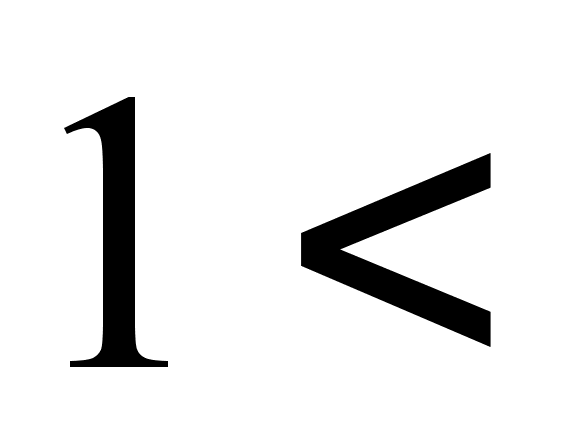(and back). This signified that eccentricity (e) of the orbit is appeared as the quantum parameter depended on (p, T). Into the atom and into its nucleus acted only exactly balanced coulomb’s and gravity forces of differently () orientations. Therefore, so-called «nuclei ‘s forces» are not existing. The circle or the straight-line motions in Nature are impossible in order to a really curvature in space and time. But as natural indicator of this curvature may be namely the form of body’s trajectory.

3.The real body’s trajectories in Nature

The explanation 2. It was shown in the exp. 1 for the orbital body: W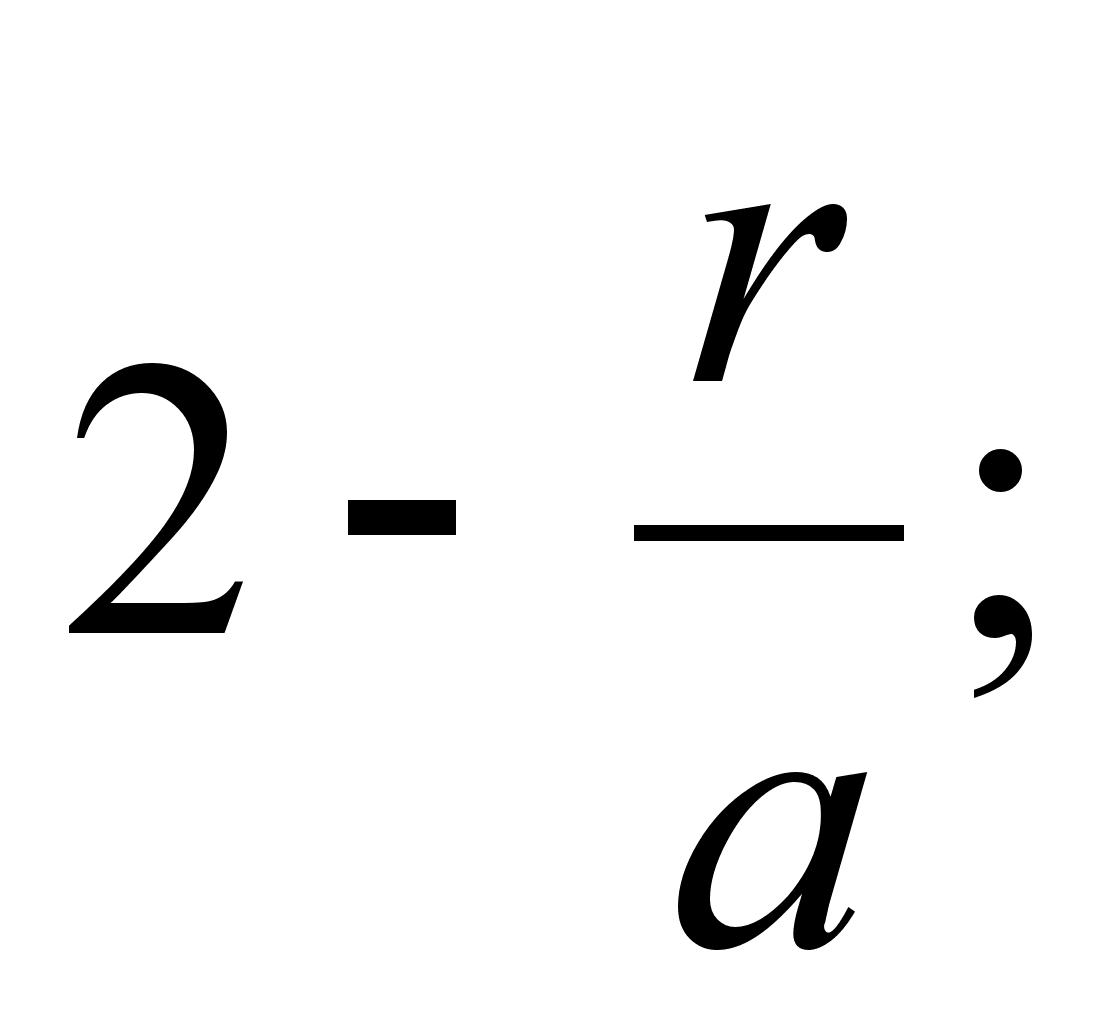for the non-orbital body: W>0,Write down the conical equation (for the circle, ellipse, parabola, hyperbola) in the non-dimensional forms

- for the ellipse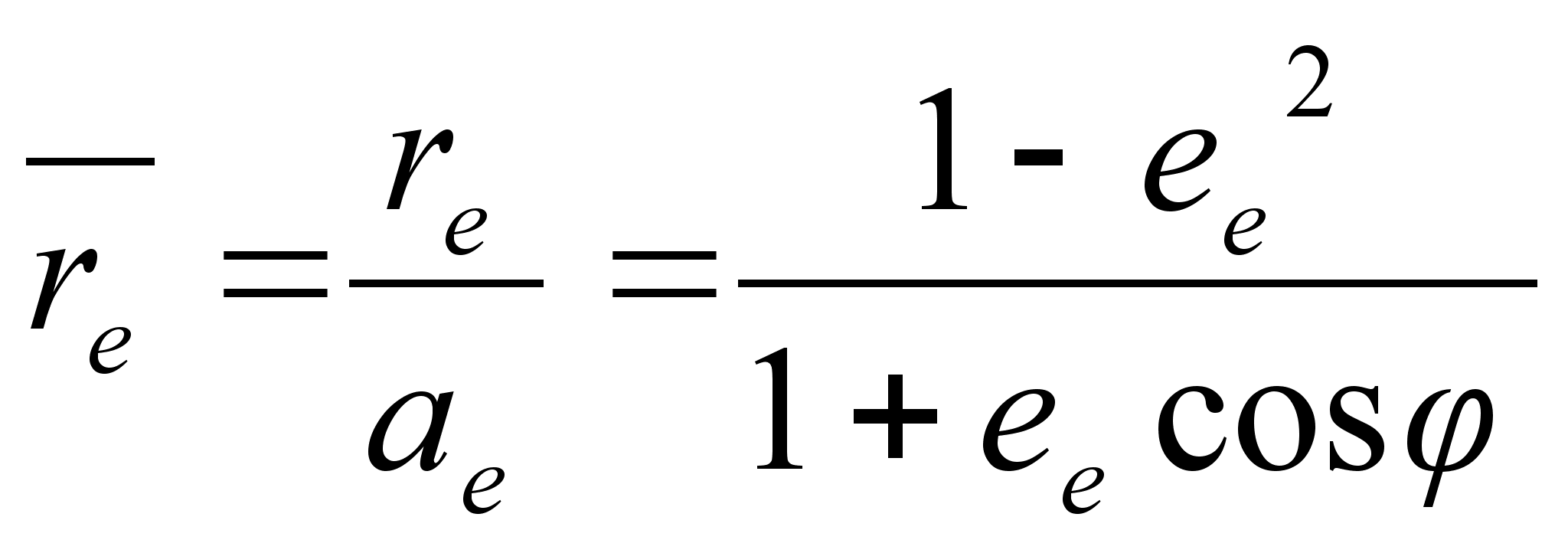, where 0, (2)

- for the hyperbola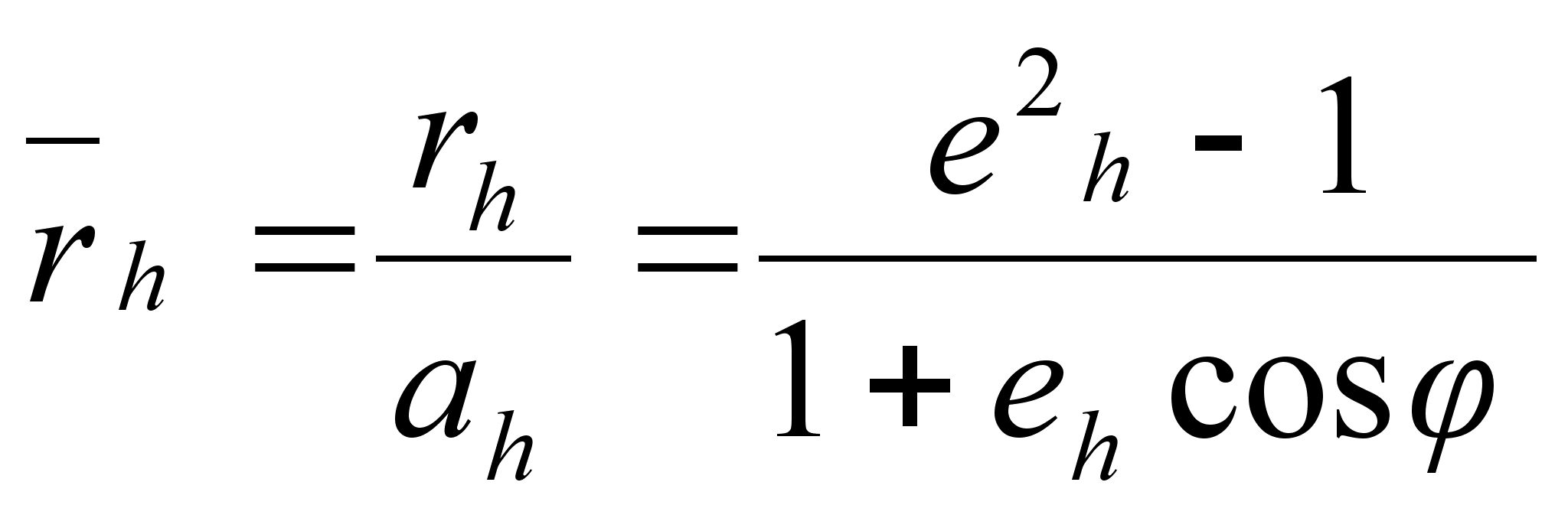, where 1. (3)

By means of (2), (3),(A) we obtain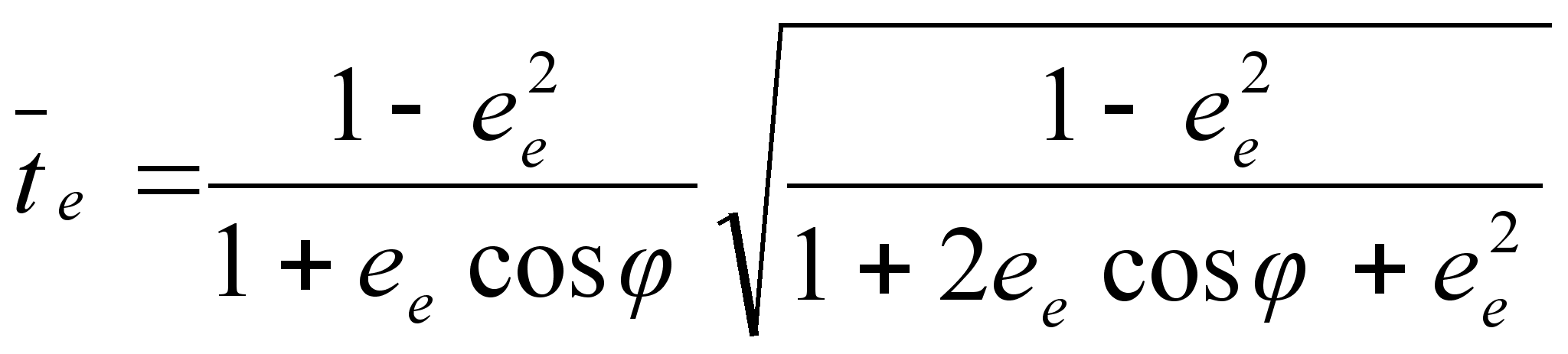, where, (a)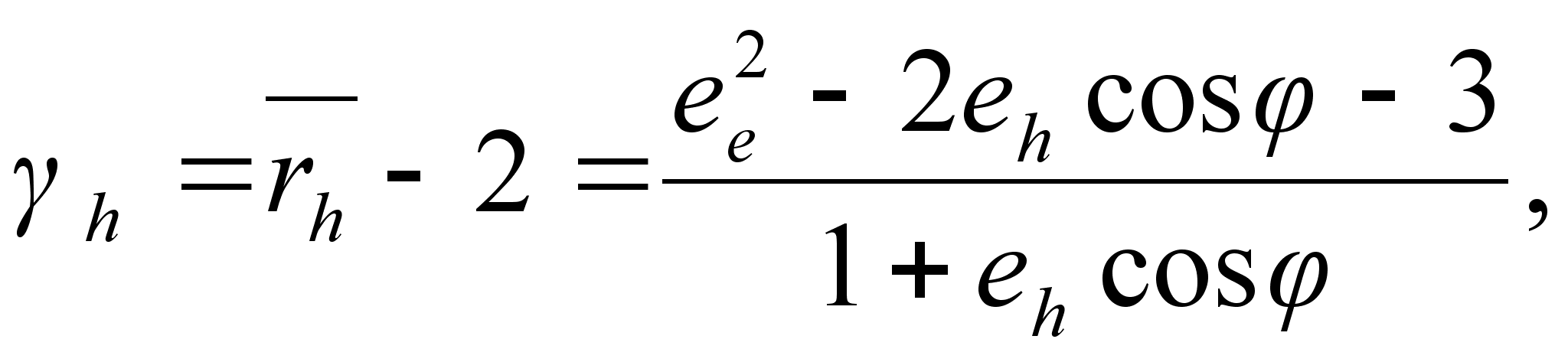, where(b)

For the straight-line: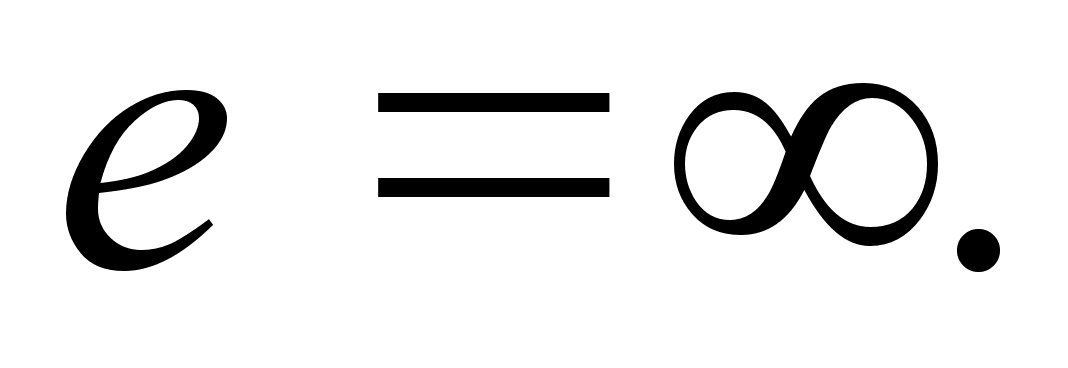Therefore,(c). For the circle:. Therefore,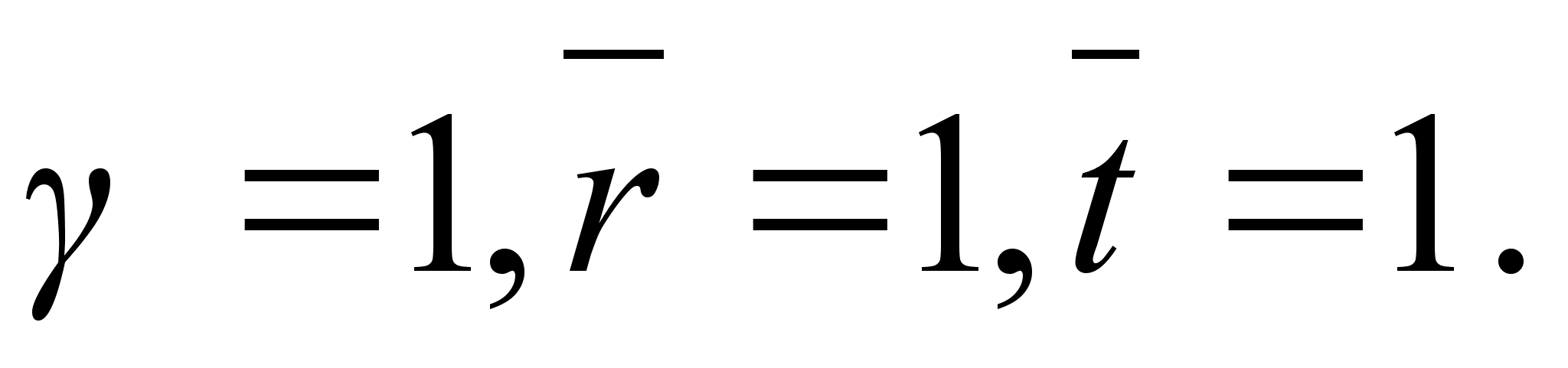(d)

For the parabola:Therefore,(e). But for the elliptical and hyperbolical trajectories parameters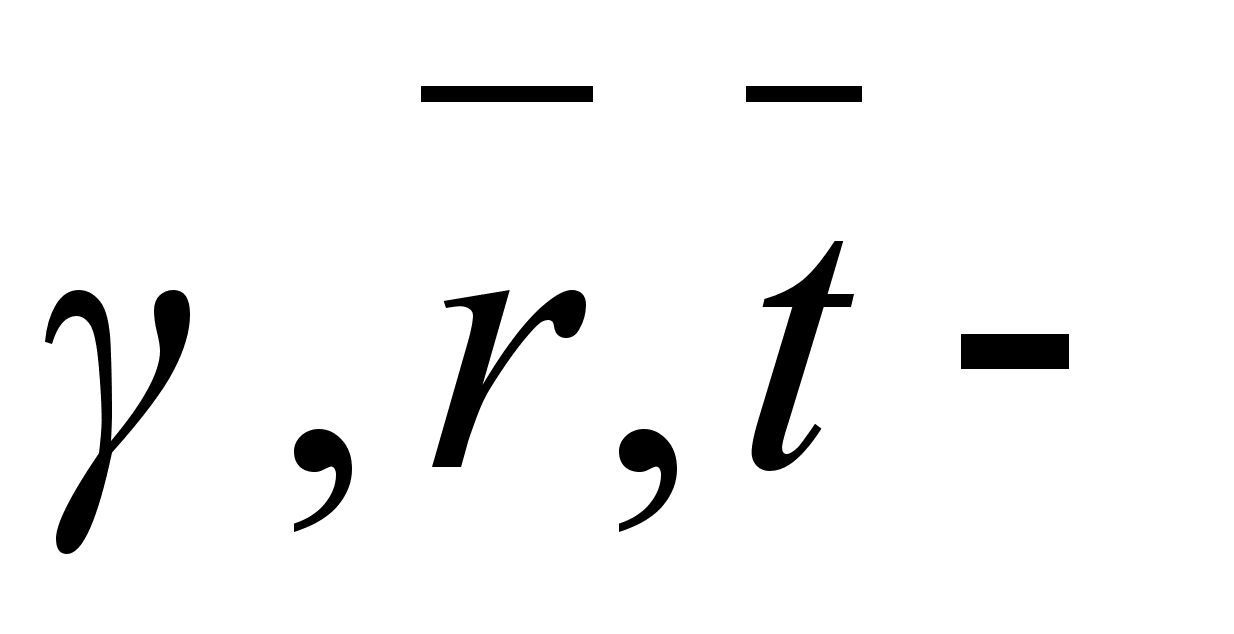are periodical ones.

4.Some useful formulas

Cephler’s the Third law has the next modern form (where f – the gravity constant, M – mass of the Sun,- the orbit ‘s semi-axis,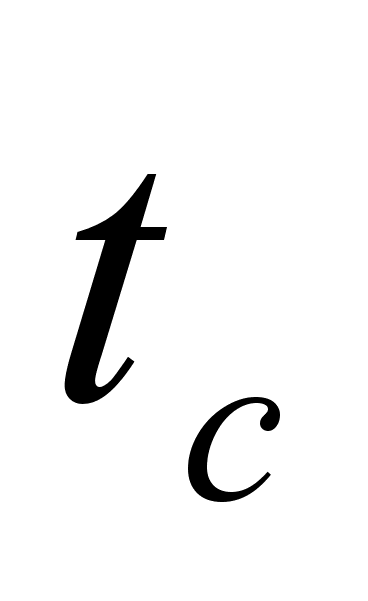– period)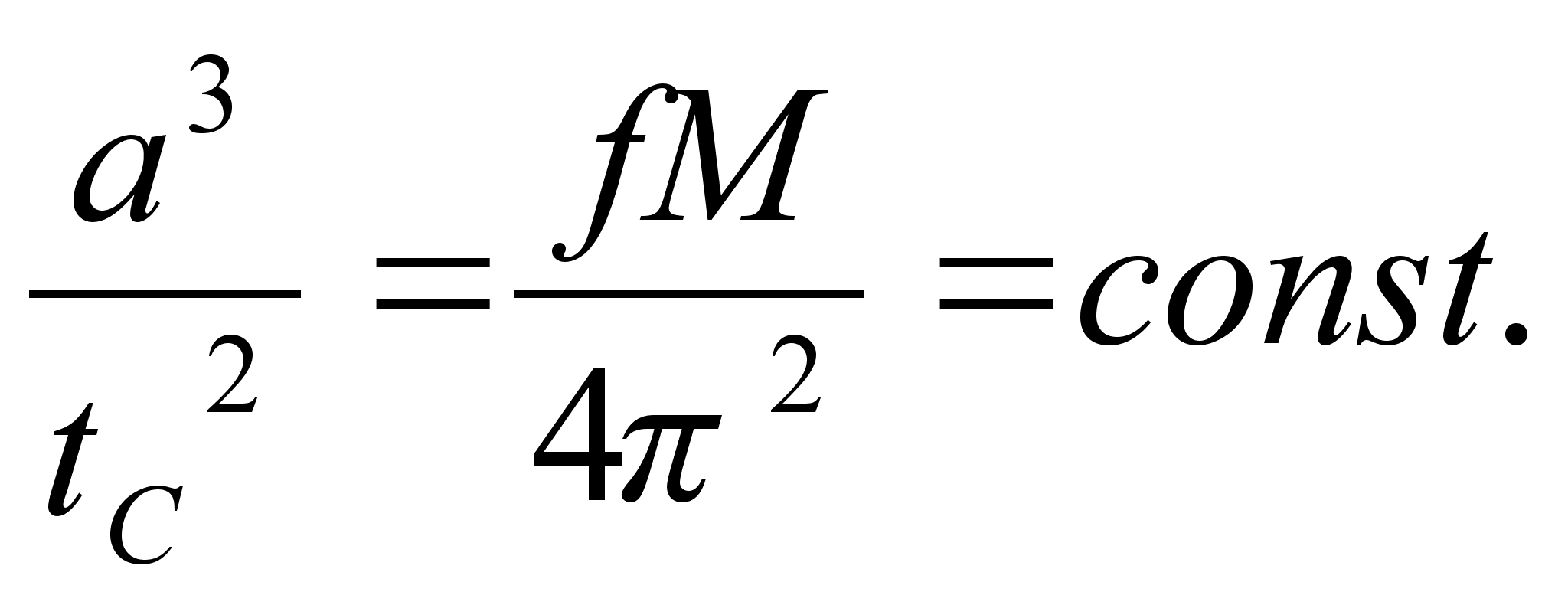But as w=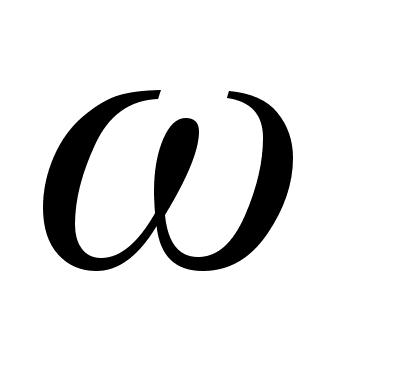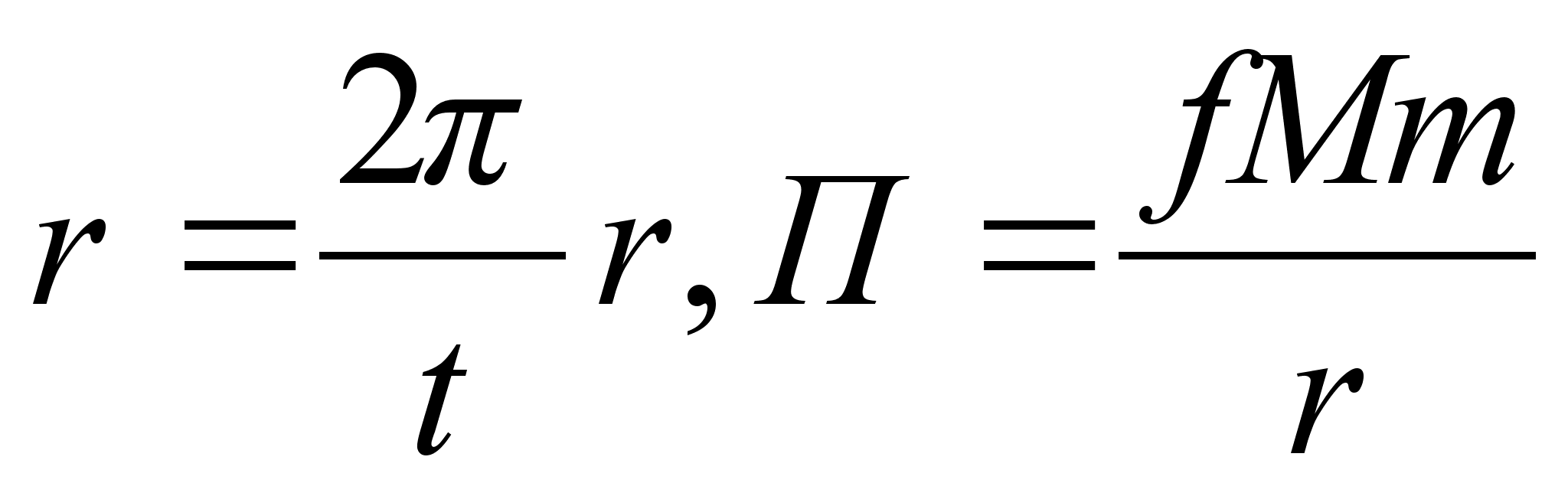(where m – the planet’s mass) we obtain from (1)From these equations we obtain the correlation (A), where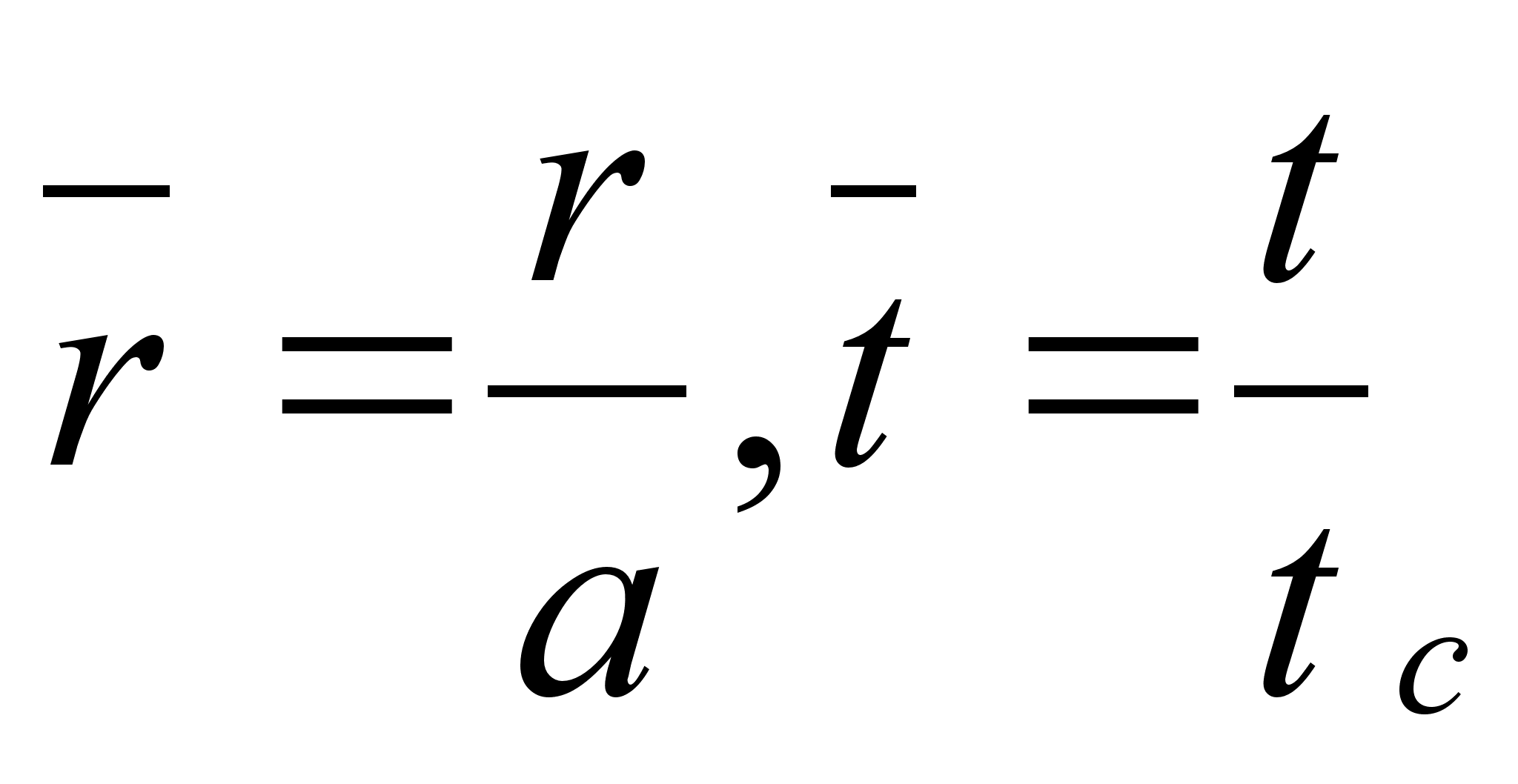. Also from the (1) we obtain: a)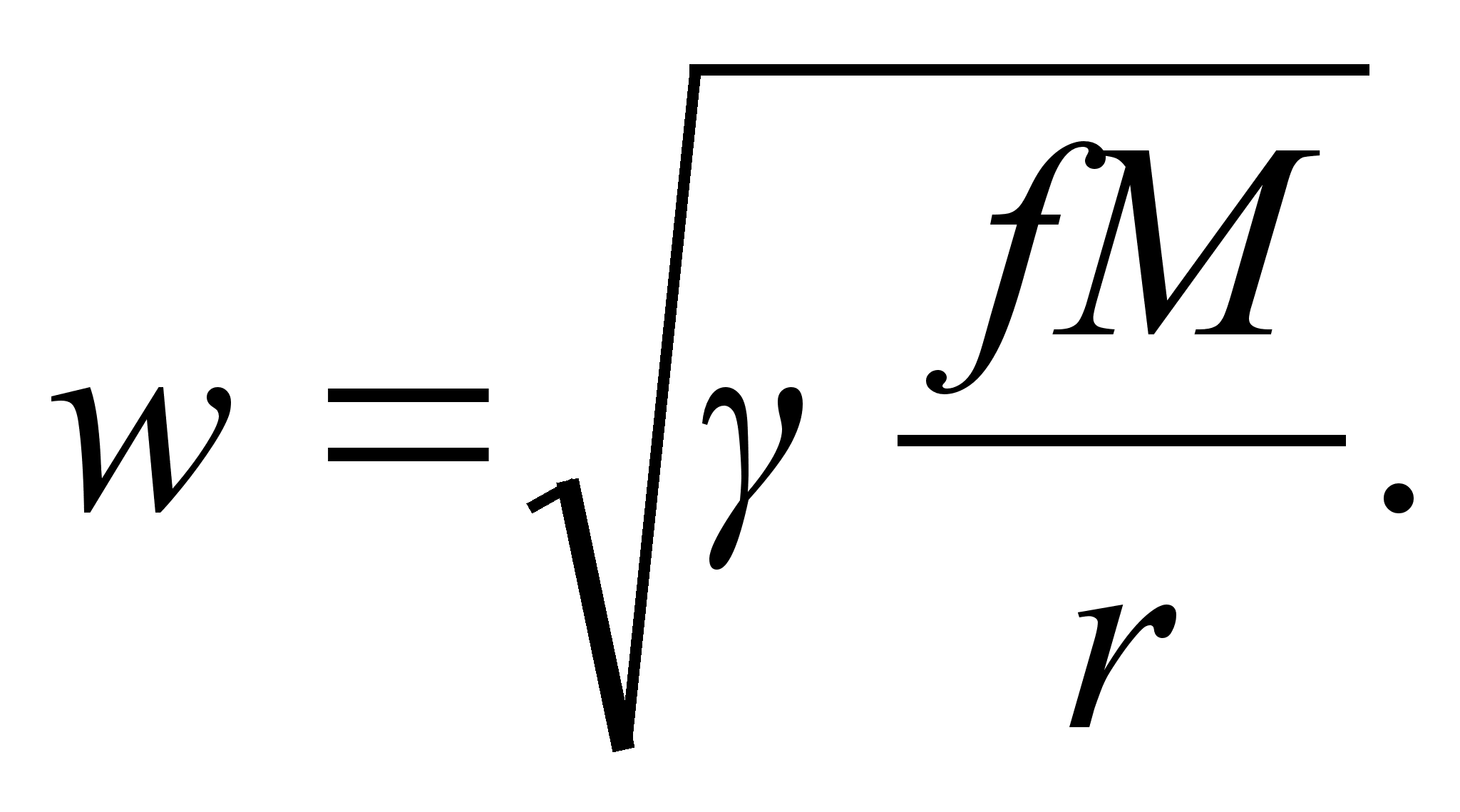For the circle () motion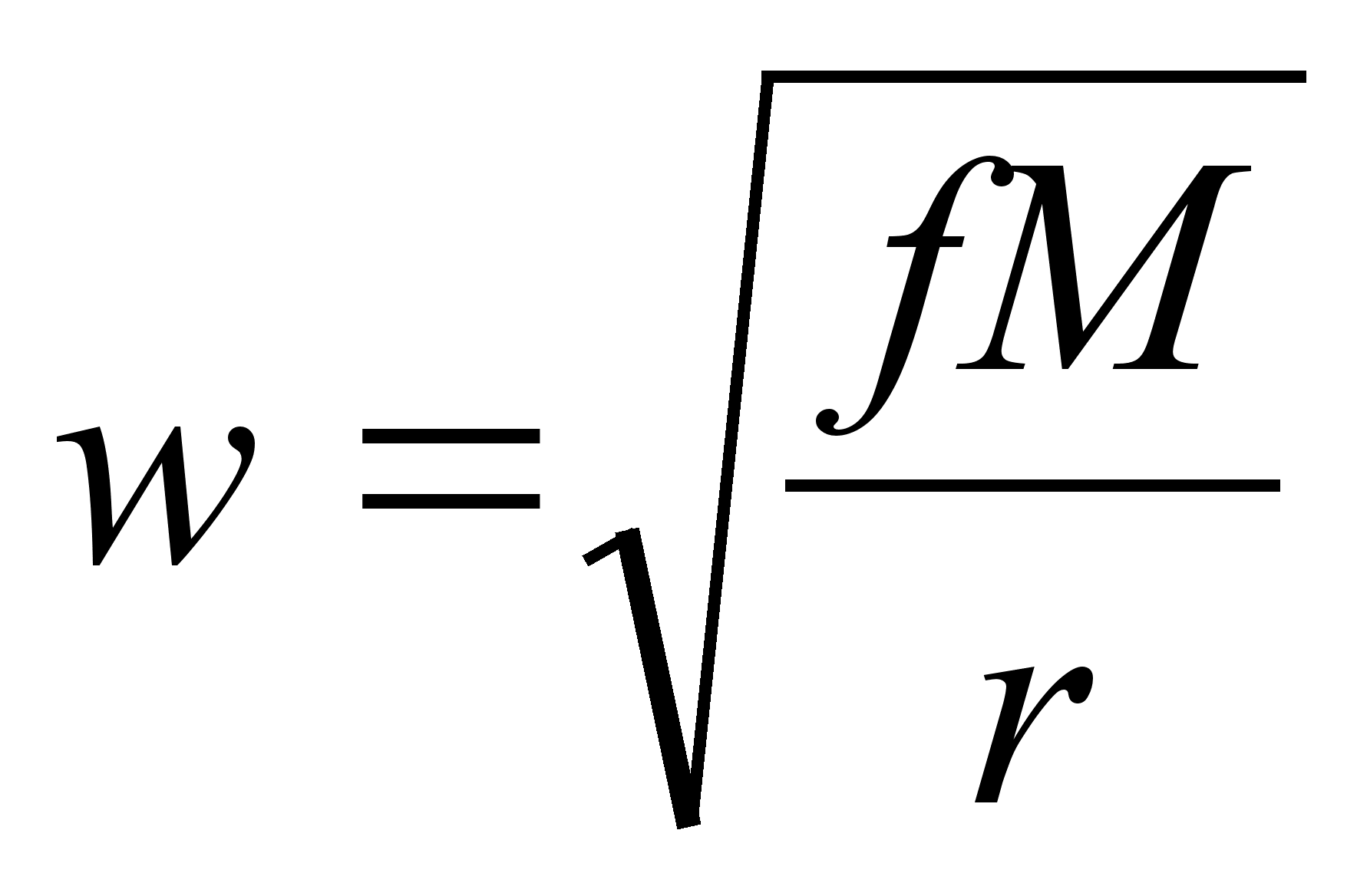- the first cosmic velocity. For the parabolic () motion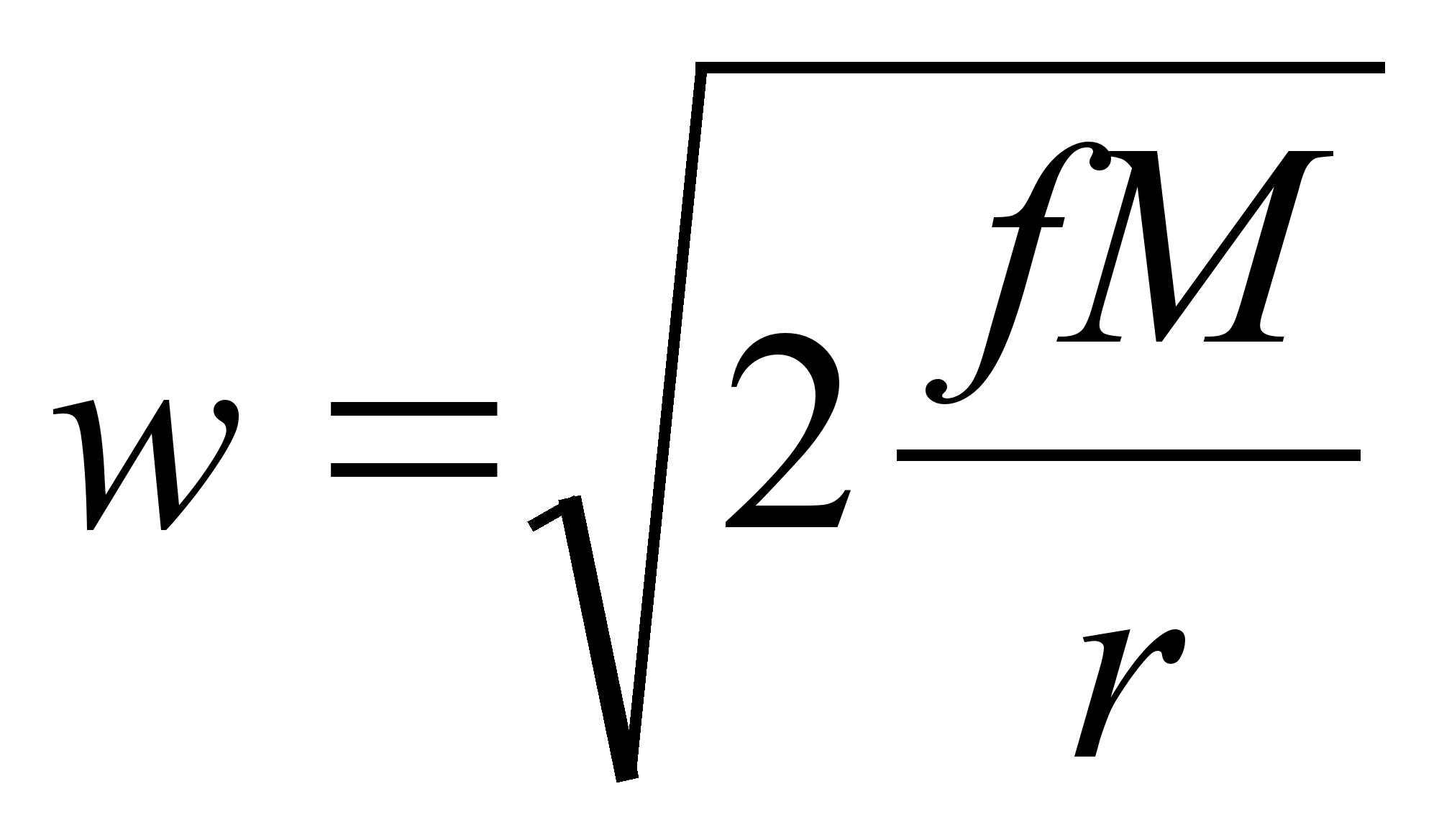- the second (or so-called - the «parabolic») cosmic velocity, and etc. b)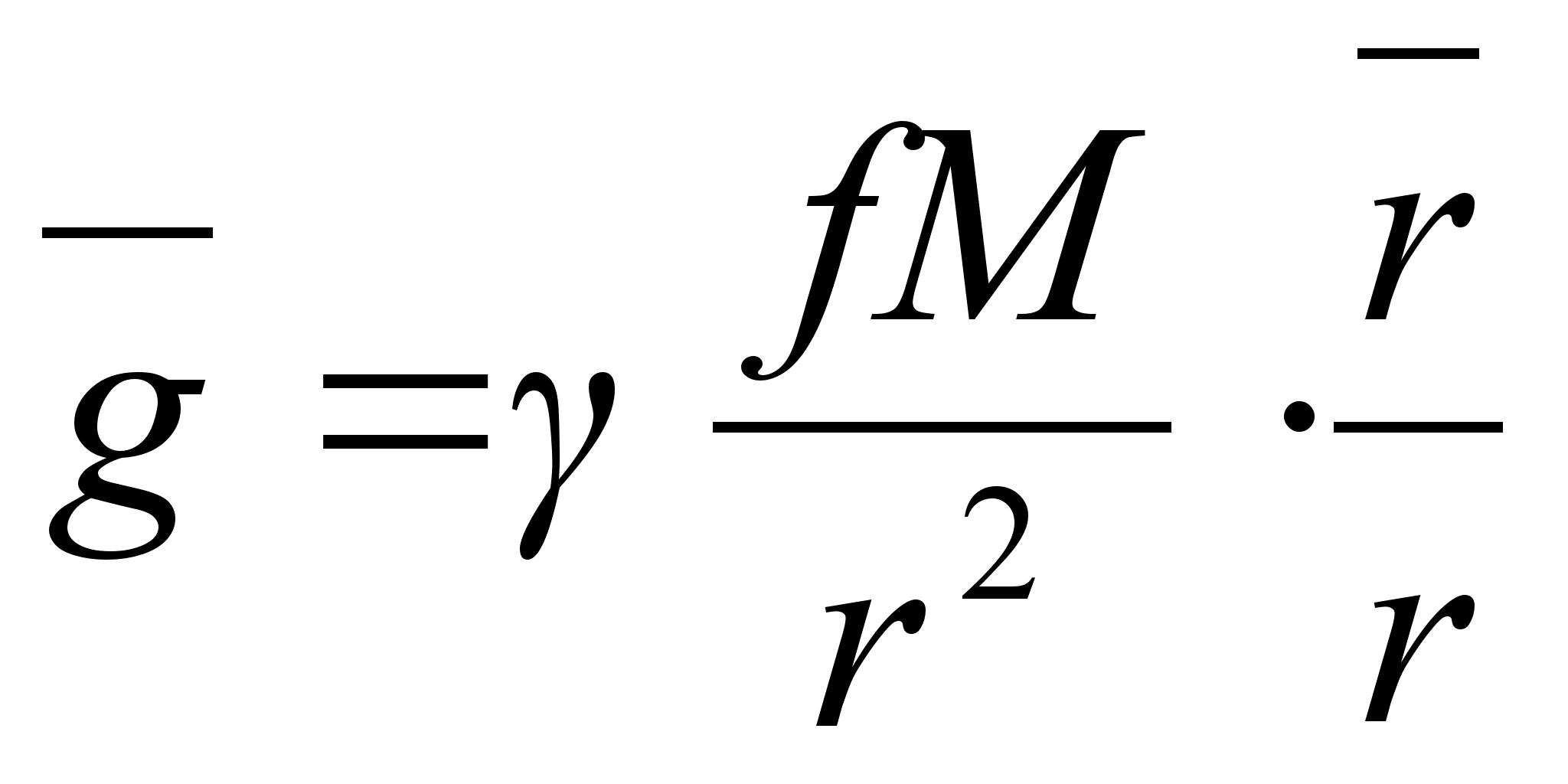(where M – the planet’s mass, r–the planet’s radius,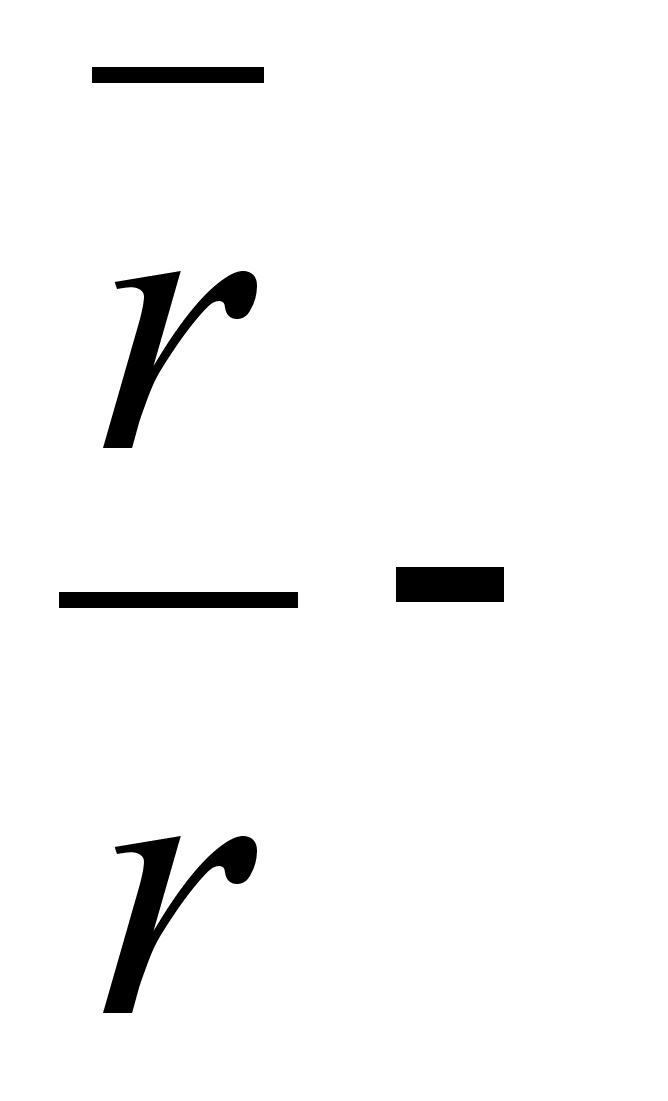the basis vector). Therefore, the body’s weight () on the planet depended on the form its orbit. For the circle () motion, we obtain:- the acceleration of gravity. From the (B’) for the photon (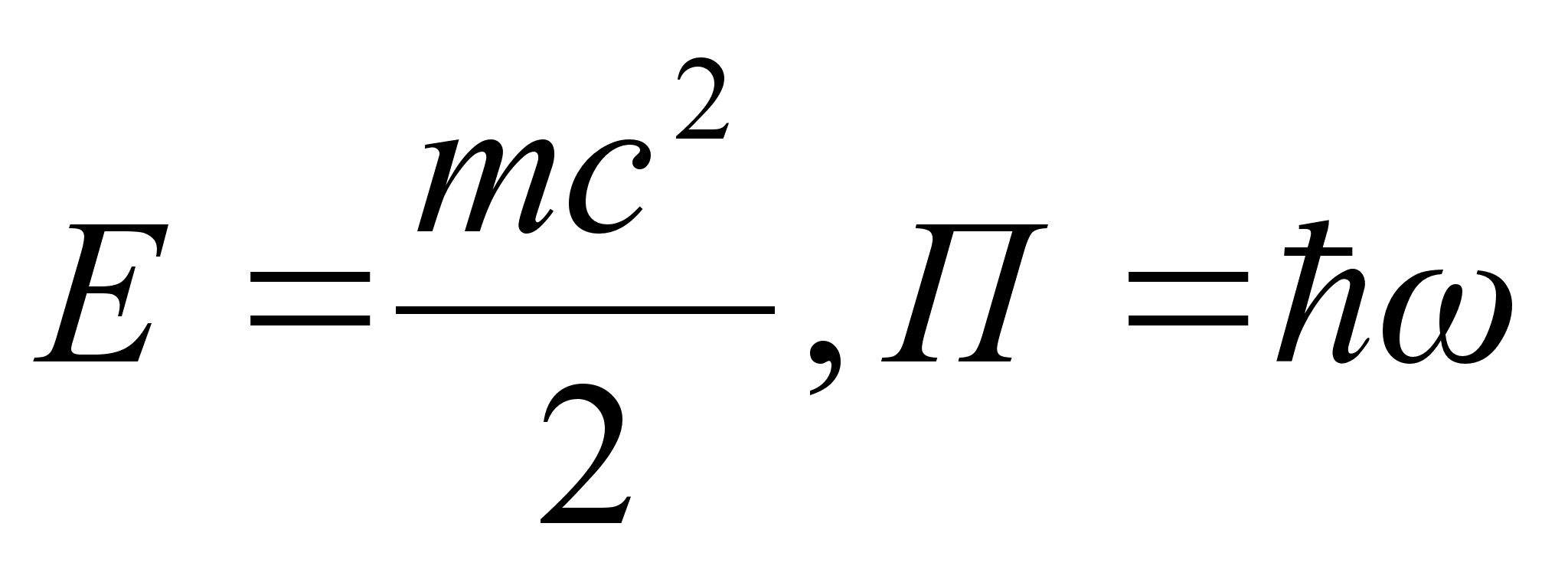) , we obtain :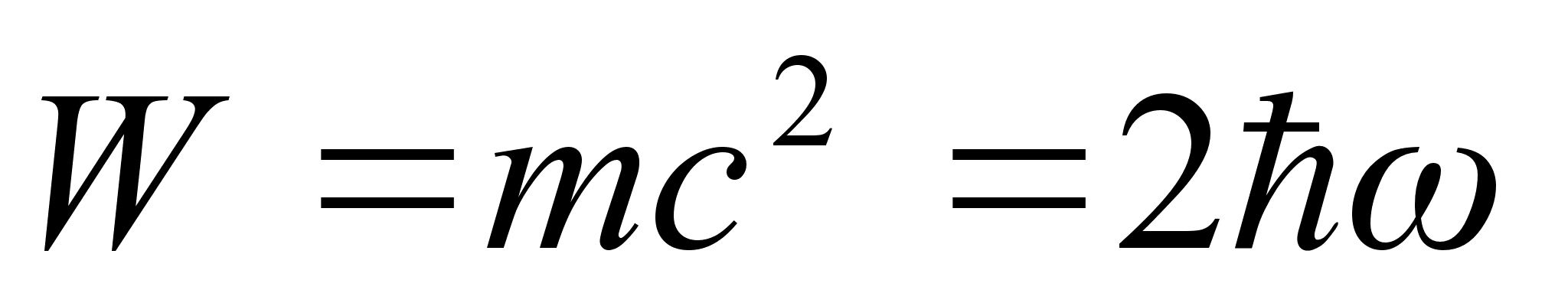- the full photon’s energy. And etc. You will be certained soon about the parameter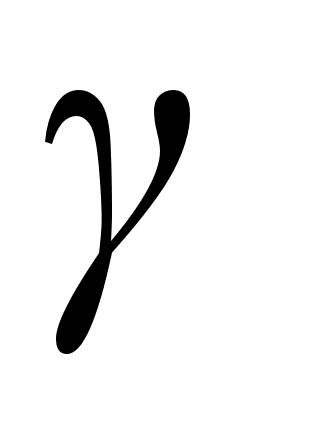fundamental significance in thermomechanics of macro- and microcosm.

Thank you. To the next report in March, 08. G.I.G.© 2012 Рефераты, доклады, дипломные и курсовые работы.